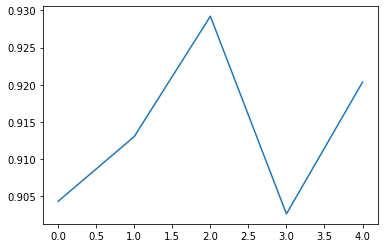# Neural Network for Classification

## Goal¶

This post aims to introduce (shallow) neural network for classification using scikit-learn.

Reference

## Libraries¶

In :
import pandas as pd
import numpy as np
from sklearn.neural_network import MLPClassifier
from sklearn.model_selection import cross_val_score
import matplotlib.pyplot as plt
%matplotlib inline


In :
breast_cancer = load_breast_cancer()
df_breast_cancer = pd.DataFrame(breast_cancer['data'], columns=breast_cancer['feature_names'])
df_breast_cancer['target'] = breast_cancer['target']


Out:
mean radius mean texture mean perimeter mean area mean smoothness mean compactness mean concavity mean concave points mean symmetry mean fractal dimension ... worst texture worst perimeter worst area worst smoothness worst compactness worst concavity worst concave points worst symmetry worst fractal dimension target
0 17.99 10.38 122.80 1001.0 0.11840 0.27760 0.3001 0.14710 0.2419 0.07871 ... 17.33 184.60 2019.0 0.1622 0.6656 0.7119 0.2654 0.4601 0.11890 0
1 20.57 17.77 132.90 1326.0 0.08474 0.07864 0.0869 0.07017 0.1812 0.05667 ... 23.41 158.80 1956.0 0.1238 0.1866 0.2416 0.1860 0.2750 0.08902 0
2 19.69 21.25 130.00 1203.0 0.10960 0.15990 0.1974 0.12790 0.2069 0.05999 ... 25.53 152.50 1709.0 0.1444 0.4245 0.4504 0.2430 0.3613 0.08758 0
3 11.42 20.38 77.58 386.1 0.14250 0.28390 0.2414 0.10520 0.2597 0.09744 ... 26.50 98.87 567.7 0.2098 0.8663 0.6869 0.2575 0.6638 0.17300 0
4 20.29 14.34 135.10 1297.0 0.10030 0.13280 0.1980 0.10430 0.1809 0.05883 ... 16.67 152.20 1575.0 0.1374 0.2050 0.4000 0.1625 0.2364 0.07678 0

5 rows × 31 columns

## Create Neural Network¶

In :
clf = MLPClassifier(solver='lbfgs', alpha=1e-5,
hidden_layer_sizes=(10,3,3), random_state=1)


In :
cv_score = cross_val_score(clf,
X=df_breast_cancer.iloc[:, :-1],
y=df_breast_cancer['target'],
cv=5)
plt.plot(cv_score);